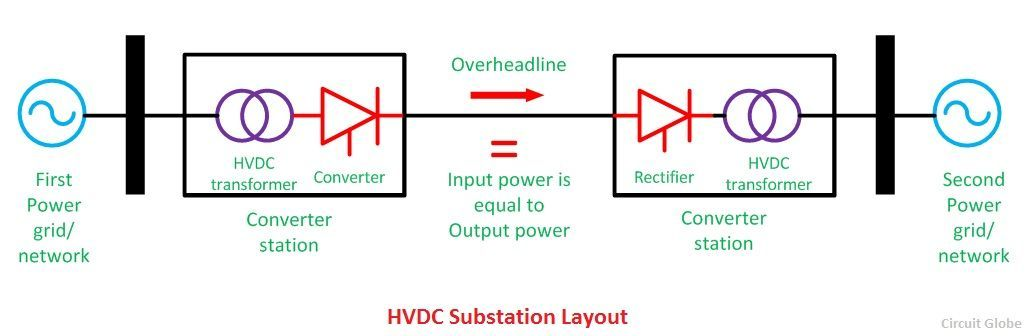# HVDC Transmission

Definition: High voltage direct current (HVDC) power systems use D.C. for transmission of bulk power over long distances. For long-distance power transmission, HVDC lines are less expensive, and losses are less as compared to AC transmission. It interconnects the networks that have different frequencies and characteristics.

In AC transmission, alternating waves of voltage and current travels in the line which change its direction every millisecond; due to which losses occur in the form of heat. Unlike AC lines, the voltage and current waves don’t change their direction in DC. HVDC lines increase the efficiency of transmission lines due to which power is rapidly transferred.

In a combined AC and DC system, generated AC voltage is converted into DC at the sending end. Then, the DC voltage is inverted to AC at the receiving end, for distribution purposes. Thus, the conversion and inversion equipment are also needed at the two ends of the line. HVDC transmission is economical only for long distance transmission lines having a length more than 600kms and for underground cables of length more than 50kms.

## How does HVDC transmission system work?

In generating substation, AC power is generated which can be converted into DC by using a rectifier. In HVDC substation or converter substation rectifiers and inverters are placed at both the ends of a line. The rectifier terminal changes the AC to DC, while the inverter terminal converts DC to AC.

The DC is flowing with the overhead lines and at the user end again DC is converted into AC by using inverters, which are placed in converter substation. The power remains the same at the sending and receiving ends of the line. DC is transmitted over long distances because it decreases the losses and improves the efficiency.A system having more than two converter stations and one transmission line is called a ‘two terminal DC system’ or a ‘point-to-point system’. Similarly, if substation has more than two converter stations and interconnecting DC terminal lines, it is called multiterminal DC substation.

### Economic Distance For HVDC transmission lines

DC lines are cheaper than the AC lines, but the cost of DC terminal equipment is very high as compared to AC terminal cables (shown in the graph below). Thus, the initial cost is high in HVDC transmission system, and it is low in the AC system.The point where two curves meet is called the breakeven distance. Above the breakeven distance, the HVDC system becomes cheaper. Breakeven distance changes from 500 to 900 km in overhead transmission lines.

1.  A lesser number of conductors and insulators are required thereby reducing the cost of the overall system.
2. It requires less phase to phase and ground to ground clearance.
3. Their towers are less costly and cheaper.
4. Lesser corona loss is less as compared to HVAC transmission lines of similar power.
5. Power loss is reduced with DC because fewer numbers of lines are required for power transmission.
6. The HVDC system uses earth return. If any fault occurs in one pole, the other pole with ‘earth returns’ behaves like an independent circuit. This results in a more flexible system.
7. The HVDC has the asynchronous connection between two AC stations connected through an HVDC link; i.e., the transmission of power is independent of sending frequencies to receiving end frequencies. Hence, it interconnects two substations with different frequencies.
8. Due to the absence of frequency in the HVDC line, losses like skin effect and proximity effect does not occur in the system.
9. It does not generate or absorb any reactive power. So, there is no need for reactive power compensation.
10. The very accurate and lossless power flows through DC link.

1. Converter substations are placed at both the sending and the receiving end of the transmission lines, which result in increasing the cost.
2. Inverter and rectifier terminals generate harmonics which can be reduced by using active filters which are also very expensive.
3. If a fault occurs in the AC substation, it may result in a power failure for the HVDC substation placed near to it
4. Inverter used in Converter substations have limited overload capacity.
5. Circuit breakers are used in HVDC for circuit breaking, which is also very expensive.
6. It does not have transformers for changing the voltage levels.
7. Heat loss occurs in converter substation, which has to be reduced by using the active cooling system.
8. HVDC link itself is also very complicated.

### Conclusion

Considering all the advantages of DC, it seems that HVDC lines are more proficient than AC lines. But, the initial cost of HVDC substation is very high and their substation equipment is quite complicated.Thus, for long distance transmission it is preferable that power is generated in AC, and for transmission, it is converted into DC and then again converted back into AC for final use. This system is economical and also improves the efficiency of the system.